# Reconstruction of potential function for Sturm-Liouville operator with Coulomb potential

## Abstract

In this paper, we are concerned with an inverse problem for the Sturm-Liouville operator with Coulomb potential using a new kind of spectral data that is known as nodal points. We give a reconstruction of q as a limit of a sequence of functions whose n th term is dependent only on eigenvalue and its associated nodal data. It is mentioned that this method is based on the works of Law and Yang, but we have applied the method to the singular Sturm-Liouville problem.

MSC:34L05, 45C05.

## 1 Introduction

Inverse problems of spectral analysis imply the reconstruction of a linear operator from some or other of its spectral characteristics. Such characteristics are spectra (for different boundary conditions), normalizing constants, spectral functions, scattering data, etc. An early important result in this direction, which gave vital impetus for further development of inverse problem theory, was obtained in . At present, inverse problems are studied for certain special classes of ordinary differential operators. Inverse problems from two spectra are the most simple in their formulation and well studied in [2, 3]. An effective method of constructing a regular and singular Sturm-Liouville operator from a spectral function or from two spectra is given in .

We note that the details of the inverse problem for singular equations are given in the monographs  and references therein.

In some recent interesting works [12, 13], Hald and McLaughlin and Browne and Sleeman have taken a new approach to inverse spectral theory for the Sturm-Liouville problem. The novelty of these works lies in the use of nodal points as the given spectral data. In recent years, inverse nodal problems have been studied by several authors etc.

In this paper, we deal with an inverse nodal problem for the Sturm-Liouville operator with Coulomb potential. We have reconstructed the potential function q from the nodal points of eigenfunctions, provided q is smooth enough. The method is based on a series of works by Law and Yang [14, 17].

Before giving the main results, we mention some physical properties of the Sturm-Liouville operator with Coulomb potential. Learning about the motion of electrons moving under the Coulomb potential is of significance in quantum theory. Solving these types of problems allows us to find energy levels not only for a hydrogen atom but also for single valence electron atoms such as sodium. For hydrogen atom, the Coulomb potential is given by $U=\frac{-{e}^{2}}{r}$, where r is the radius of the nucleus, e is electronic charge. According to this, we use the time-dependent Schrödinger equation

$iħ\frac{\partial \mathrm{\Psi }}{\partial t}=-\frac{{ħ}^{2}}{2m}\frac{{\partial }^{2}\mathrm{\Psi }}{\partial {x}^{2}}+U\left(x,y,z\right)\mathrm{\Psi },\phantom{\rule{2em}{0ex}}{\int }_{{R}^{3}}{|\mathrm{\Psi }|}^{2}\phantom{\rule{0.2em}{0ex}}dx\phantom{\rule{0.2em}{0ex}}dy\phantom{\rule{0.2em}{0ex}}dz=1,$

where Ψ is the wave function, ħ is Planck’s constant and m is the mass of electron. In this equation, if the Fourier transform is applied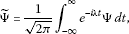it will convert to energy equation dependent on the situation as follows:

$\frac{{ħ}^{2}}{2m}{▽}^{2}\stackrel{˜}{\mathrm{\Psi }}+\stackrel{˜}{U}\stackrel{˜}{\mathrm{\Psi }}=E\stackrel{˜}{\mathrm{\Psi }}.$

Therefore, energy equation in the field with the Coulomb potential becomes

$-\frac{{ħ}^{2}}{2m}{▽}^{2}\stackrel{˜}{\mathrm{\Psi }}+\left(E+\frac{{e}^{2}}{r}\right)\stackrel{˜}{\mathrm{\Psi }}=0.$

If this hydrogen atom is substituted to other potential area, then the energy equation becomes

$-\frac{{ħ}^{2}}{2m}{▽}^{2}\stackrel{˜}{\mathrm{\Psi }}+\left(E+\frac{{e}^{2}}{r}+q\left(x,y,z\right)\right)\stackrel{˜}{\mathrm{\Psi }}=0.$

If we make the necessary transformation, then we can get a Sturm-Liouville equation with Coulomb potential

$-{y}^{\mathrm{\prime }\mathrm{\prime }}+\left[\frac{A}{x}+q\left(x\right)\right]y=\lambda y,$

where λ is a parameter which corresponds to the energy .

We consider the singular Sturm-Liouville problem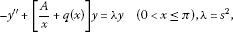(1.1)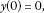(1.2)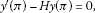(1.3)

in which the function $q\left(x\right)\in {L}^{1}\left[0,\pi \right]$, A, H are finite numbers and $\frac{y\left(x\right)}{x}\in C\left[0,\pi \right]$. Next, we denote by $\phi \left(x,s\right)$ the solution of (1.1) satisfying the initial condition

$\phi \left(0,s\right)=0,\phantom{\rule{2em}{0ex}}{\phi }^{\mathrm{\prime }}\left(0,s\right)=s.$
(1.4)

Let ${\lambda }_{n}$ be the n th eigenvalue and $0<{x}_{1}^{n}<{x}_{2}^{n}<\cdots <{x}_{i}^{n}<\pi$, $i=1,2,\dots ,n-1$ be nodal points of the n th eigenfunction. Also, let ${I}_{i}^{n}=\left[{x}_{i}^{n},{x}_{i+1}^{n}\right]$ be the i th nodal domain of the n th eigenfunction and let ${l}_{i}^{n}=|{l}_{i}^{n}|={x}_{i+1}^{n}-{x}_{i}^{n}$ be the associated nodal length. We also define the function ${j}_{n}\left(x\right)$ by ${j}_{n}\left(x\right)=max\left\{i:{x}_{i}^{n}.

## 2 Main results

In this section, we try to obtain some asymptotic results and a reconstruction formula for the potential q, which has been obtained as a solution of an inverse nodal problem.

Lemma 2.1 The solution of problem (1.1)-(1.3) has the following form:

$\phi \left(x,s\right)=sinsx+{\int }_{0}^{x}\frac{sins\left(x-t\right)}{s}\left\{\frac{A}{t}+q\left(t\right)\right\}\phi \left(t,s\right)\phantom{\rule{0.2em}{0ex}}dt,$
(2.1)

where $\frac{\phi \left(t,s\right)}{t}\in C\left[0,\pi \right]$.

Proof Because $\phi \left(x,s\right)$ satisfies equation (1.1), we getBy integrating the first term twice on the right-hand side by parts and taking the conditions into account (1.2), we find that

$\phi \left(x,s\right)=sinsx+{\int }_{0}^{x}\frac{sins\left(x-t\right)}{s}\left\{\frac{A}{t}+q\left(t\right)\right\}\phi \left(t,s\right)\phantom{\rule{0.2em}{0ex}}dt,$

where $\frac{\phi \left(t,s\right)}{t}\in C\left[0,\pi \right]$. □

Lemma 2.2 The eigenvalues of problem (1.1)-1.3) are the roots of (1.3). This spectral characteristic satisfies the following asymptotic expression :

${s}_{n}=\sqrt{{\lambda }_{n}}=n+\frac{1}{2}+\frac{A}{2\pi }\frac{ln\left(n+\frac{1}{2}\right)}{\left(n+\frac{1}{2}\right)}+\frac{{c}_{0}}{\left(n+\frac{1}{2}\right)}+O\left(\frac{lnn}{{n}^{2}}\right),$
(2.2)

whereLemma 2.3 Assume that $q\in {L}^{1}\left(0,\pi \right)$. Then, as $n\to \mathrm{\infty }$,(2.3)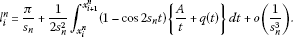(2.4)

Proof By using some iterations and trigonometric calculations in (2.1), we obtain

$\begin{array}{rcl}\phi \left(x,s\right)& =& sinsx+\frac{sinsx}{2s}{\int }_{0}^{x}sin2st\left\{\frac{A}{t}+q\left(t\right)\right\}\phantom{\rule{0.2em}{0ex}}dt\\ -\frac{cossx}{2s}{\int }_{0}^{x}\left(1-cos2st\right)\left\{\frac{A}{t}+q\left(t\right)\right\}\phantom{\rule{0.2em}{0ex}}dt+o\left(\frac{1}{{s}^{2}}\right).\end{array}$

If $\phi \left(x,s\right)$ is equal to zero and $cos\lambda x$ is not close to zero, then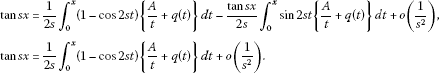Now, we take $s={s}_{n}$ and $x={x}_{i}^{n}$. Because Taylor’s expansion for the arctangent function is given by

$arctanx=\pi i-\sum _{k=0}^{\mathrm{\infty }}\frac{{\left(-1\right)}^{k+1}{x}^{2k+1}}{2k+1}$

for some integer i, then

${s}_{n}{x}_{i}^{n}=\pi i+\frac{1}{2{s}_{n}}{\int }_{0}^{{x}_{i}^{n}}\left(1-cos2st\right)\left\{\frac{A}{t}+q\left(t\right)\right\}\phantom{\rule{0.2em}{0ex}}dt+o\left(\frac{1}{{s}_{n}^{2}}\right).$

Therefore

${x}_{i}^{n}=\frac{\pi i}{{s}_{n}}+\frac{1}{2{s}_{n}^{2}}{\int }_{0}^{{x}_{i}^{n}}\left(1-cos2st\right)\left\{\frac{A}{t}+q\left(t\right)\right\}\phantom{\rule{0.2em}{0ex}}dt+o\left(\frac{1}{{s}_{n}^{3}}\right).$

The nodal length is

${l}_{i}^{n}={x}_{i+1}^{n}-{x}_{i}^{n},\phantom{\rule{1em}{0ex}}\phantom{\rule{1em}{0ex}}{l}_{i}^{n}=\frac{\pi }{{s}_{n}}+\frac{1}{2{s}_{n}^{2}}{\int }_{{x}_{i}^{n}}^{{x}_{i+1}^{n}}\left(1-cos2st\right)\left\{\frac{A}{t}+q\left(t\right)\right\}\phantom{\rule{0.2em}{0ex}}dt+o\left(\frac{1}{{s}_{n}^{3}}\right).$

This completes the proof of Lemma 2.3. □

Lemma 2.4 Suppose $f\in {L}^{1}\left(0,\pi \right)$. Then, for almost every $x\in \left(0,\pi \right)$ with $j={j}_{n}\left(x\right)$,

$\underset{n\to \mathrm{\infty }}{lim}\frac{{s}_{n}}{\pi }{\int }_{{x}_{j}^{n}}^{{x}_{j+1}^{n}}f\left(t\right)\phantom{\rule{0.2em}{0ex}}dt=f\left(x\right).$

Proof Since $f\in {L}^{1}\left(0,\pi \right)$, $\frac{d}{dx}{\int }_{a}^{x}f\left(t\right)\phantom{\rule{0.2em}{0ex}}dt=f\left(x\right)$ almost everywhere. Thus, given any $\zeta >0$, when n is sufficiently large and for almost every $x\in \left(0,\pi \right)$,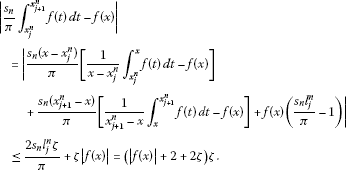This proves Lemma 2.4. □

Theorem 2.1 The potential function $q\left(x\right)\in {L}^{1}\left(0,\pi \right)$ satisfies

$q\left(x\right)=\underset{n\to \mathrm{\infty }}{lim}\left[2{s}_{n}^{2}\left(\frac{{s}_{n}{l}_{j}^{n}}{\pi }-1\right)-{s}_{n}Aln\left(\frac{{x}_{j+1}^{n}}{{x}_{j}^{n}}\right)+\frac{{s}_{n}A}{\pi }{\int }_{{x}_{j}^{n}}^{{x}_{j+1}^{n}}\frac{cos2{s}_{n}t}{t}\phantom{\rule{0.2em}{0ex}}dt\right]$

for almost every $x\in \left(0,\pi \right)$ with $j={j}_{n}\left(x\right)$. We note that the asymptotic expression for ${s}_{n}$ in Theorem 2.1 implies that $q\left(x\right)={lim}_{n\to \mathrm{\infty }}{F}_{n}\left(x\right)$.

Proof When we consider (2.4) in the form

${l}_{j}^{n}=\frac{\pi }{{s}_{n}}+\frac{1}{2{s}_{n}^{2}}{\int }_{{x}_{j}^{n}}^{{x}_{j+1}^{n}}\left(1-cos2st\right)\left\{\frac{A}{t}+q\left(t\right)\right\}\phantom{\rule{0.2em}{0ex}}dt+o\left(\frac{1}{{s}_{n}^{3}}\right)$

so that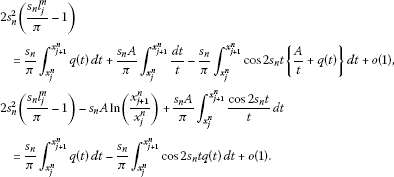By Lemma 2.4

$\underset{n\to \mathrm{\infty }}{lim}\frac{{s}_{n}}{\pi }{\int }_{{x}_{j}^{n}}^{{x}_{j+1}^{n}}q\left(t\right)\phantom{\rule{0.2em}{0ex}}dt=q\left(x\right)$

for almost every $x\in \left(0,\pi \right)$.

It remains to show that for almost every $x\in \left(0,\pi \right)$,

${T}_{n}\left(x\right):=\frac{{s}_{n}}{\pi }{\int }_{{x}_{j}^{n}}^{{x}_{j+1}^{n}}cos2{s}_{n}tq\left(t\right)\phantom{\rule{0.2em}{0ex}}dt$

tends to zero as $n\to \mathrm{\infty }$. Take a sequence of continuous functions ${q}_{k}$ which converges to q in ${L}^{1}\left(0,\pi \right)$. Then ${q}_{k}$ has a subsequence converging to q almost everywhere in $\left(0,\pi \right)$. We call this subsequence ${q}_{k}$. Take any x such that ${q}_{k}\left(x\right)$ converges to $q\left(x\right)$. Then for a given $\epsilon >0$, we can fix a large k such that $|{q}_{k}\left(x\right)-q\left(x\right)|<\epsilon$. Hence

$\begin{array}{rcl}{T}_{n}\left(x\right)& =& \frac{{s}_{n}}{\pi }{\int }_{{x}_{j}^{n}}^{{x}_{j+1}^{n}}cos2{s}_{n}t\left[q\left(t\right)-{q}_{k}\left(t\right)\right]\phantom{\rule{0.2em}{0ex}}dt+\frac{{s}_{n}}{\pi }{\int }_{{x}_{j}^{n}}^{{x}_{j+1}^{n}}cos2{s}_{n}t\left[{q}_{k}\left(t\right)-{q}_{k}\left(x\right)\right]\phantom{\rule{0.2em}{0ex}}dt\\ +\frac{{s}_{n}}{\pi }{\int }_{{x}_{j}^{n}}^{{x}_{j+1}^{n}}cos2{s}_{n}t{q}_{k}\left(x\right)\phantom{\rule{0.2em}{0ex}}dt\\ =& {A}_{n}+{B}_{n}+{C}_{n}.\end{array}$

By Lemma 2.3,

${C}_{n}=\frac{{q}_{k}\left(x\right)}{2\pi }\left[sin\left(2{s}_{n}{x}_{j+1}^{n}\right)-sin\left(2{s}_{n}{x}_{j}^{n}\right)\right]={q}_{k}\left(x\right)O\left(\frac{1}{n}\right)$

and so it tends to zero as $n\to \mathrm{\infty }$. By Lemma 2.4, the first term ${A}_{n}$ satisfies, when n is sufficiently large,

$\begin{array}{rcl}|{A}_{n}|& =& |\frac{{s}_{n}}{\pi }{\int }_{{x}_{j}^{n}}^{{x}_{j+1}^{n}}cos2{s}_{n}t\left[q\left(t\right)-{q}_{k}\left(t\right)\right]\phantom{\rule{0.2em}{0ex}}dt|\\ \le & \frac{{s}_{n}}{\pi }{\int }_{{x}_{j}^{n}}^{{x}_{j+1}^{n}}|q\left(t\right)-{q}_{k}\left(t\right)|\phantom{\rule{0.2em}{0ex}}dt\\ <& |q\left(x\right)-{q}_{k}\left(x\right)|+\epsilon \\ <& 2\epsilon .\end{array}$

On the other hand,

$|{B}_{n}|=|\frac{{s}_{n}}{\pi }{\int }_{{x}_{j}^{n}}^{{x}_{j+1}^{n}}cos2{s}_{n}t\left[{q}_{k}\left(t\right)-{q}_{k}\left(x\right)\right]\phantom{\rule{0.2em}{0ex}}dt|\le \frac{{s}_{n}}{\pi }{\int }_{{x}_{j}^{n}}^{{x}_{j+1}^{n}}|{q}_{k}\left(t\right)-{q}_{k}\left(x\right)|\phantom{\rule{0.2em}{0ex}}dt.$

Because ${q}_{k}$ is continuous, this term is arbitrarily every $x\in \left(0,\pi \right)$. Hence we conclude that ${lim}_{n\to \mathrm{\infty }}{T}_{n}\left(x\right)=0$. This proves Theorem 2.1. □

Lemma 2.5 We take a sequence ${f}_{k}\in C\left[0,\pi \right]$ converges to $f\in {L}^{1}$, then, for any large enough n, with $j={j}_{n}\left(x\right)$ as $k\to \mathrm{\infty }$

${\parallel \frac{{s}_{n}}{\pi }{\int }_{{x}_{j}^{n}}^{{x}_{j+1}^{n}}\left[{f}_{k}\left(t\right)-f\left(t\right)\right]\phantom{\rule{0.2em}{0ex}}dt\parallel }_{1}\to 0.$

Proof By (2.4) and observation that the integral ${\int }_{{x}_{j}^{n}}^{{x}_{j+1}^{n}}\left[{f}_{k}\left(t\right)-f\left(t\right)\right]\phantom{\rule{0.2em}{0ex}}dt$ is constant on any nodal interval ${I}_{j}^{n}$, we obtain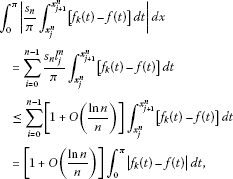and for $k\to \mathrm{\infty }$ this term converges to zero. □

Lemma 2.6 Suppose that $q\in {L}^{1}\left(0,\pi \right)$, then as $n\to \mathrm{\infty }$ with $j={j}_{n}\left(x\right)$,

${\parallel \frac{{s}_{n}}{\pi }{\int }_{{x}_{j}^{n}}^{{x}_{j+1}^{n}}q\left(t\right)\phantom{\rule{0.2em}{0ex}}dt-q\left(x\right)\parallel }_{1}\to 0.$

Proof Firstly, let us show that if q is continuous on $\left[0,\pi \right]$, the result is satisfied. Let $M={max}_{x\in \left[0,\pi \right]}|q\left(x\right)|$. By using the intermediate value theorem, there exists $\xi \in \left(a,x\right)$ such that

$|\frac{1}{x-a}{\int }_{a}^{x}q\left(t\right)\phantom{\rule{0.2em}{0ex}}dt-q\left(x\right)|=|q\left(\xi \right)-q\left(x\right)|.$

If x is close enough to a, the difference can be arbitrarily small. Then, for all $\epsilon >0$, when n is large enough, with $j={j}_{n}\left(x\right)$ we get

$\begin{array}{rcl}|\frac{{s}_{n}}{\pi }{\int }_{{x}_{j}^{n}}^{{x}_{j+1}^{n}}q\left(t\right)\phantom{\rule{0.2em}{0ex}}dt-q\left(x\right)|& \le & |\frac{{s}_{n}\left(x-{x}_{j}^{n}\right)}{\pi }\left[\frac{1}{x-{x}_{j}^{n}}{\int }_{{x}_{j}^{n}}^{x}q\left(t\right)\phantom{\rule{0.2em}{0ex}}dt-q\left(x\right)\right]|\\ +|\frac{{s}_{n}\left({x}_{j+1}^{n}-x\right)}{\pi }\left[\frac{1}{{x}_{j+1}^{n}-x}{\int }_{x}^{{x}_{j+1}^{n}}q\left(t\right)\phantom{\rule{0.2em}{0ex}}dt-q\left(x\right)\right]|\\ +|q\left(x\right)||\left(\frac{{s}_{n}{l}_{j}^{n}}{\pi }-1\right)|\\ \le & \frac{2{s}_{n}{l}_{j}^{n}\epsilon }{\pi }+M\epsilon \\ \le & \left(M+2+2\epsilon \right)\epsilon .\end{array}$

In the above process, we assume that $x\ne {x}_{j}^{n}$. The estimate also holds if $x={x}_{j}^{n}$. Hence if $q\in C\left[0,\pi \right]$, $\frac{{s}_{n}}{\pi }{\int }_{{x}_{j}^{n}}^{{x}_{j+1}^{n}}q\left(t\right)\phantom{\rule{0.2em}{0ex}}dt$ converges to $q\left(x\right)$ uniformly on $\left(0,\pi \right)$. Thus $\parallel \frac{{s}_{n}}{\pi }{\int }_{{x}_{j}^{n}}^{{x}_{j+1}^{n}}q\left(t\right)\phantom{\rule{0.2em}{0ex}}dt-q\left(x\right)\parallel$ can be arbitrarily small. Because $C\left[0,\pi \right]$ is dense in ${L}^{1}\left(0,\pi \right)$, for any $q\in {L}^{1}\left(0,\pi \right)$, there exists a sequence ${q}_{k}\in C\left[0,\pi \right]$ convergent to q in ${L}^{1}\left(0,\pi \right)$. Hence, fix n sufficiently large,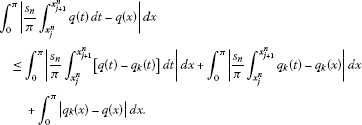From the above process and Lemma 2.5, when k is large enough, the first two terms are arbitrarily small. Hence, as $k\to \mathrm{\infty }$,

${\parallel \frac{{s}_{n}}{\pi }{\int }_{{x}_{j}^{n}}^{{x}_{j+1}^{n}}\left[{f}_{k}\left(t\right)-f\left(t\right)\right]\phantom{\rule{0.2em}{0ex}}dt\parallel }_{1}\to 0.$

□

Theorem 2.2 ${F}_{n}$ converges to q in ${L}^{1}$.

Proof When we consider the value of ${F}_{n}$, we obtain that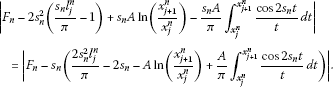It suffices to show that as $n\to \mathrm{\infty }$

${\parallel {s}_{n}\left(\frac{2{s}_{n}^{2}{l}_{j}^{n}}{\pi }-2{s}_{n}-Aln\left(\frac{{x}_{j+1}^{n}}{{x}_{j}^{n}}\right)+\frac{A}{\pi }{\int }_{{x}_{j}^{n}}^{{x}_{j+1}^{n}}\frac{cos2{s}_{n}t}{t}\phantom{\rule{0.2em}{0ex}}dt\right)-q\parallel }_{1}\to 0.$

By using (2.4) we have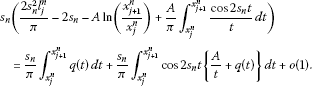Hence, we only need to prove that for $n\to \mathrm{\infty }$

${\parallel \frac{{s}_{n}}{\pi }{\int }_{{x}_{j}^{n}}^{{x}_{j+1}^{n}}q\left(t\right)\phantom{\rule{0.2em}{0ex}}dt-q\parallel }_{1}\to 0$

and

${\parallel \frac{{s}_{n}}{\pi }{\int }_{{x}_{j}^{n}}^{{x}_{j+1}^{n}}cos2{s}_{n}t\left\{\frac{A}{t}+q\left(t\right)\right\}\phantom{\rule{0.2em}{0ex}}dt\parallel }_{1}\to 0.$
(2.5)

From Lemma 2.6, the first limit holds and the second limit also holds. On the other hand, the sequence of functions

${c}_{n}\left(x\right)=\frac{{s}_{n}}{\pi }{\int }_{{x}_{j}^{n}}^{{x}_{j+1}^{n}}cos2{s}_{n}t\left\{\frac{A}{t}+q\left(t\right)\right\}\phantom{\rule{0.2em}{0ex}}dt$

converges to 0 for almost every $x\in \left(0,\pi \right)$. Furthermore,

$|{c}_{n}\left(x\right)|\le \frac{{s}_{n}}{\pi }{\int }_{{x}_{j}^{n}}^{{x}_{i+1}^{n}}|\frac{A}{t}+q\left(t\right)|\phantom{\rule{0.2em}{0ex}}dt={g}_{n}\left(x\right)$

and

${\int }_{0}^{\pi }{g}_{n}\left(x\right)\phantom{\rule{0.2em}{0ex}}dx=\sum _{i=0}^{n-1}\frac{{s}_{n}{l}_{j}^{n}}{\pi }{\int }_{{x}_{j}^{n}}^{{x}_{j+1}^{n}}|\frac{A}{t}+q\left(t\right)|\phantom{\rule{0.2em}{0ex}}dt=\left[1+O\left(\frac{lnn}{n}\right)\right]{\parallel q\parallel }_{1}.$

Then, we may apply the Lebesque dominated convergence theorem to show that (2.5) is valid. The proof of Theorem 2.2 is completed. □

## References

1. Ambartsumyan VA: Über eine frage der eigenwerttheorie. Z. Phys. 1929, 53: 690-695. 10.1007/BF01330827

2. Levitan BM: On the determination of the Sturm-Liouville operator from one and two spectra. Math. USSR, Izv. 1978, 12: 179-193. 10.1070/IM1978v012n01ABEH001844

3. Isaacson EL, Trubowitz E: The inverse Sturm-Liouville problem. I. Commun. Pure Appl. Math. 1983, 36: 767-783. 10.1002/cpa.3160360604

4. Gelfand IM, Levitan BM: On the determination of a differential equation by its spectral function. Izv. Akad. Nauk SSSR, Ser. Mat. 1951, 15: 309-360. Ams, 253-304 (1955)

5. Hochstadt H: The inverse Sturm-Liouville problem. Commun. Pure Appl. Math. 1973, 26: 715-729. 10.1002/cpa.3160260514

6. Pöschel J, Trubowitz E: Inverse Spectral Theory. Academic Press, Boston; 1987.

7. Rundell W, Sack EP: Reconstruction of a radially symmetric potential from two spectral sequences. J. Math. Anal. Appl. 2001, 264: 354-381. 10.1006/jmaa.2001.7664

8. Carlson R: Borg-Levinson theorem for Bessel operator. Pac. J. Math. 1997, 177: 1-26. 10.2140/pjm.1997.177.1

9. Chadan K, Colton D, Paivarinta L, Rundell W: An Introduction to Inverse Scattering and Inverse Spectral Problems. SIAM, Philadelphia; 1997.

10. Panakhov ES, Sat M: On the determination of the singular Sturm-Liouville operator from two spectra. Comput. Model. Eng. Sci. 2012, 84: 1-11.

11. Hald OH: Discontinuous inverse eigenvalue problem. Commun. Pure Appl. Math. 1984, 37: 539-577. 10.1002/cpa.3160370502

12. Browne PJ, Sleeman BD: Inverse nodal problems for Sturm-Liouville equation with eigenparameter dependent boundary conditions. Inverse Probl. 1996, 12: 377-381. 10.1088/0266-5611/12/4/002

13. Hald OH, McLaughlin JR: Solution of inverse nodal problems. Inverse Probl. 1989, 5: 307-347. 10.1088/0266-5611/5/3/008

14. Chen YT, Cheng YH, Law CK, Tsa J: Convergence of reconstruction formula for the potential function. Proc. Am. Math. Soc. 2002, 130: 2319-2324. 10.1090/S0002-9939-02-06297-4

15. Yang FX: A solution of the inverse nodal problem. Inverse Probl. 1997, 13: 203-213. 10.1088/0266-5611/13/1/016

16. McLaughlin JR: Inverse spectral theory using nodal points as a data - a uniqueness result. J. Differ. Equ. 1988, 73: 354-362. 10.1016/0022-0396(88)90111-8

17. Law CK, Shen CL, Yang CF: The inverse nodal problem on the smoothness of the potential function. Inverse Probl. 1999, 15: 253-263. 10.1088/0266-5611/15/1/024

18. Yurko VA, Freiling G: Inverse nodal problems for differential operators on graphs with a cycle. Tamkang J. Math. 2010, 41: 15-24.

19. Yang CF: Inverse nodal problems for the Sturm-Liouville operator with eigenparameter dependent boundary conditions. Oper. Matrices 2012, 6(1):63-77.

20. Koyunbakan H, Panakhov ES: A uniqueness theorem for inverse nodal problem. Inverse Probl. Sci. Eng. 2007, 15: 517-524. 10.1080/00423110500523143

21. Koyunbakan H: Reconstruction of potential function for diffusion operator. Numer. Funct. Anal. Optim. 2009, 30: 111-120. 10.1080/01630560802279256

22. Blohincev DI: Foundations of Quantum Mechanics. GITTL, Moscow; 1949.

23. Amirov RK, Çakmak Y, Gulyaz S: Boundary value problem for second order differential equations with Coulomb singularity on a finite interval. Indian J. Pure Appl. Math. 2006, 37: 125-140.

## Acknowledgements

The authors would like to thank the editor and referees for their valuable comments and remarks which led to a great improvement of the article.

## Author information

Authors

### Corresponding author

Correspondence to Murat Sat.

### Competing interests

The authors declare that they have no competing interests.

### Authors’ contributions

MS wrote the first draft and ESP corrected and improved the final version. Both authors read and approved the final draft.

## Rights and permissions

Reprints and Permissions

Panakhov, E.S., Sat, M. Reconstruction of potential function for Sturm-Liouville operator with Coulomb potential. Bound Value Probl 2013, 49 (2013). https://doi.org/10.1186/1687-2770-2013-49